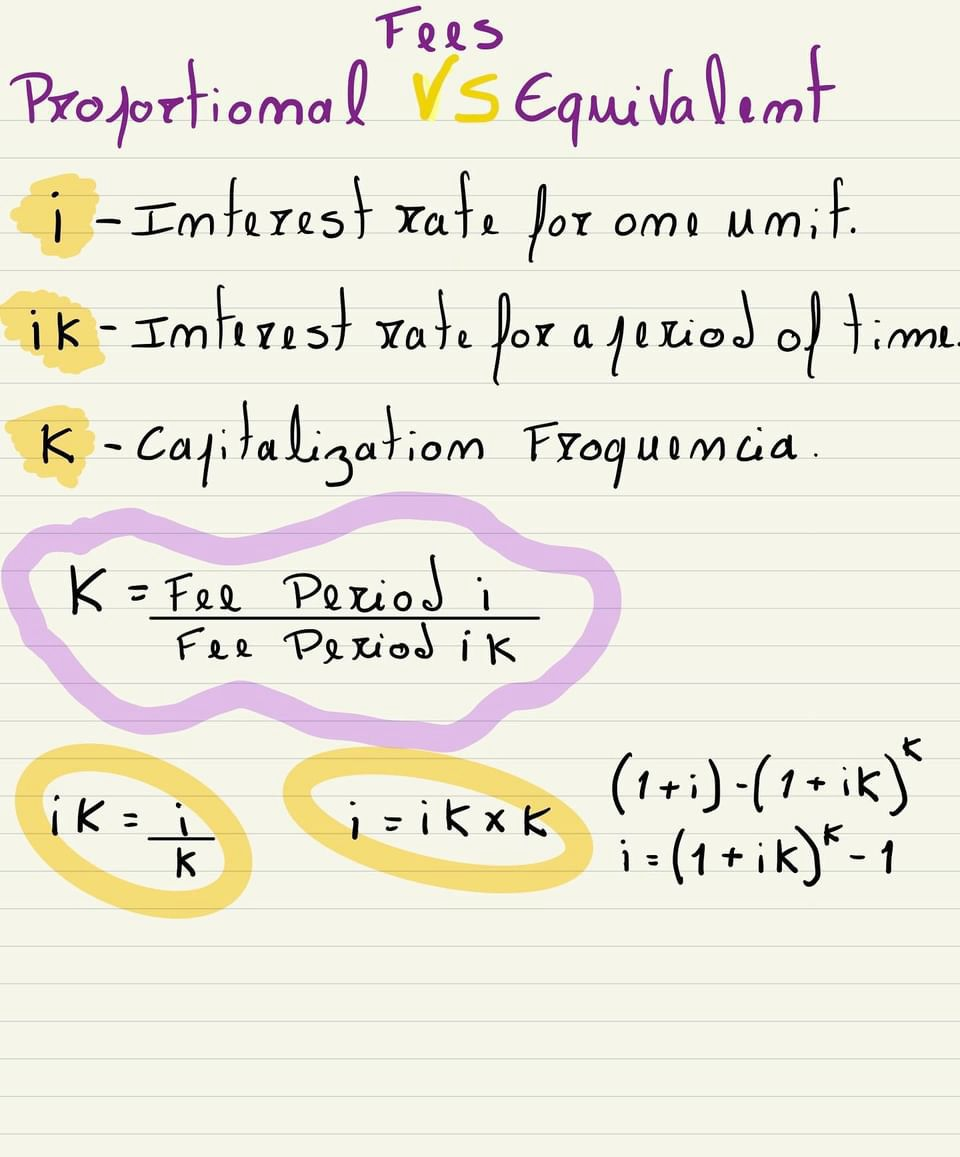Propotional VS EquivalentFees

Propotional VS Equivalent

i I-Interest rate for one unif.

ik Interest rate fox a period of time

K

- Capitalization Froquencia

K = Fee Period i

Fee Period ik

ik =

(1+i)-(1+ik)

i

i =ikxk

K

i =(1+ik)*-1

of 1